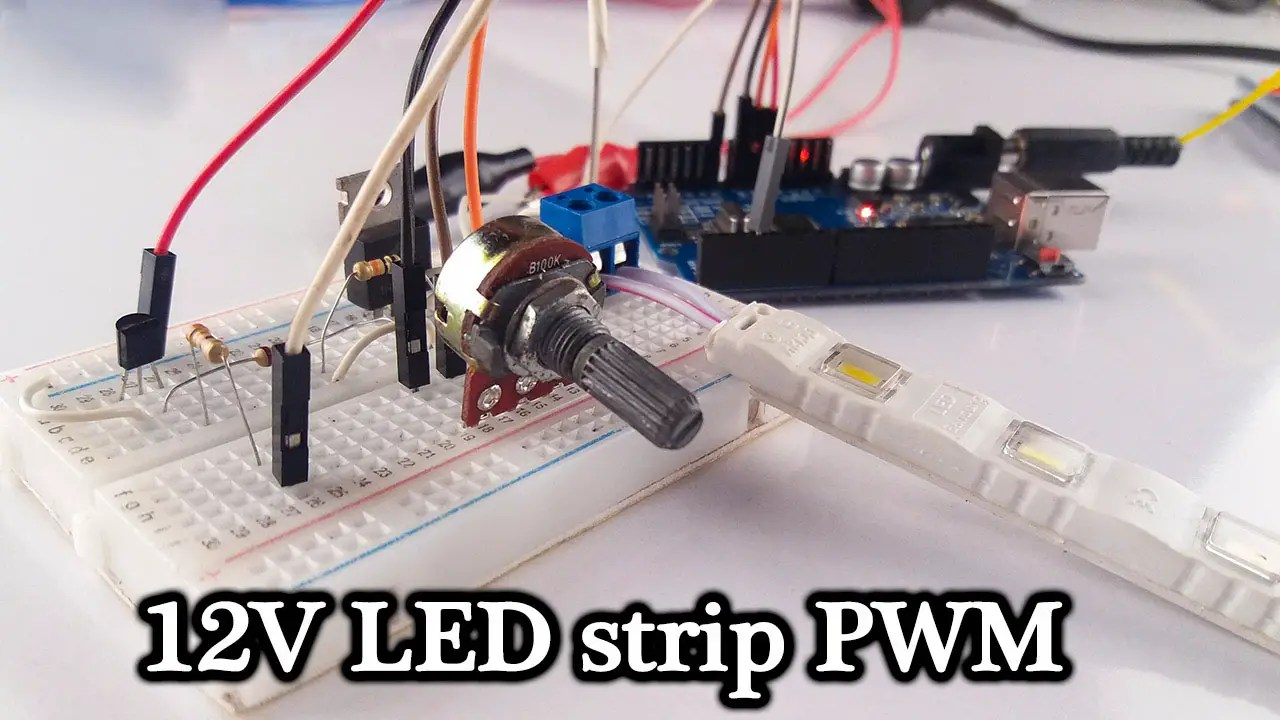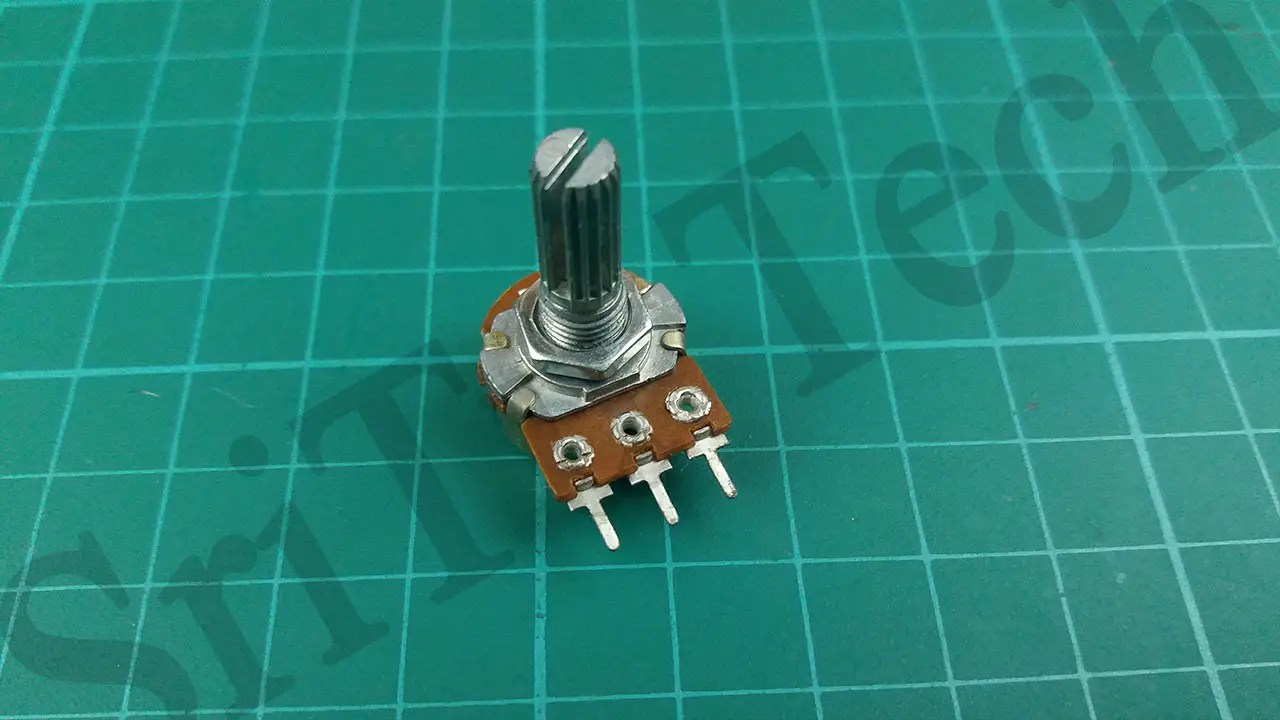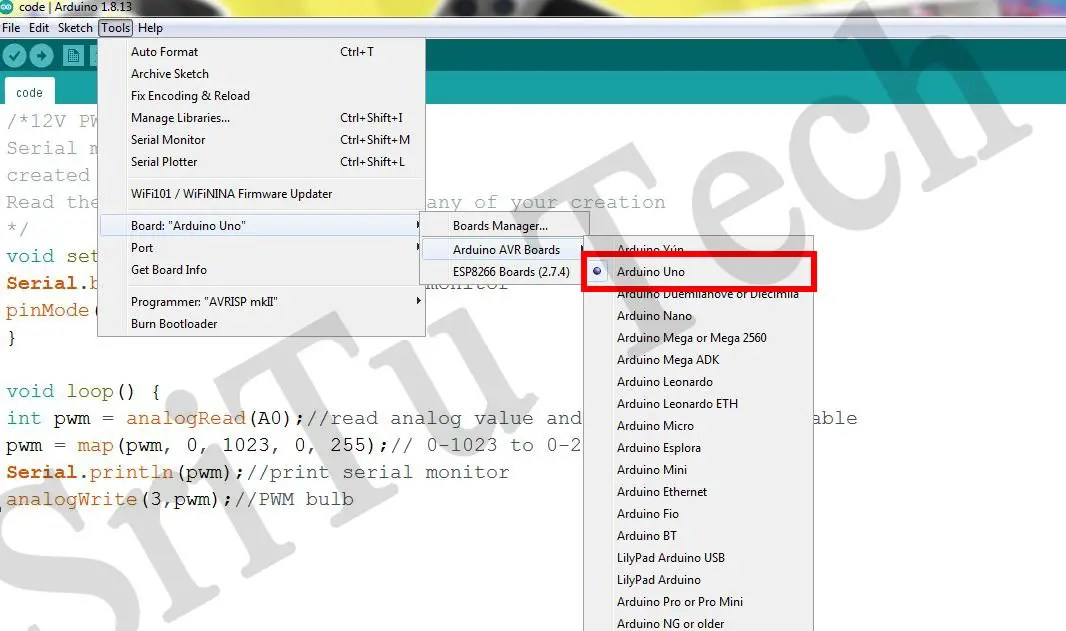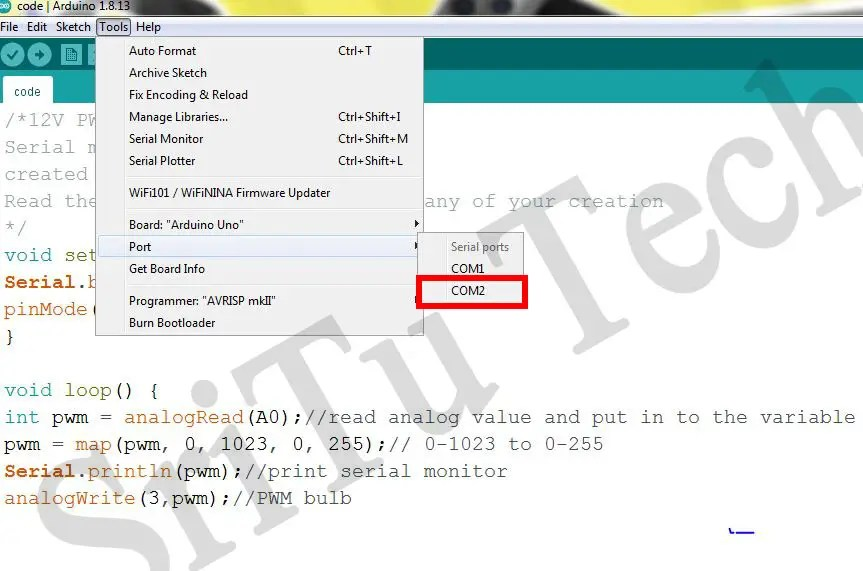# How to make a 12v PWM circuit Arduino using UNO board.

## How to make a 12v PWM circuit using ArduinoHello guys, In this tutorial, we will explain how to make a 12v PWM circuit using the Arduino platform. For this, we first need to learn what is the PWM. PWM is simply called pulse width modulation. Click this link to know more about this. So then let’s get to the project today. In this project, we will learn how to make a 12V LED strip PWM circuit using Arduino.

If you have any knowledge about Arduino, you know that we can get 5VDC voltage and 40mA current through the Arduino board. For this reason, external power must be obtained to control a 12V device. So we can do this easily through a Relay module, but we cannot get a PWM value through a Relay module. (If you do not understand Relay, click on this link)Therefore we can use a transistor for this. Through this, we can perform the PWM process properly.

So this project used a MOSFET type transistor. A transistor is simply an electronic switch. This transistor can be turned on / off to the desired size by the PWM value obtained by a PWM PIN on the Arduino board. That is, we can increase or decrease the 12v potential flowing through the transistor to the desired level. This causes the brightness of the 12v LED strip to increase or decrease. If you do not understand Transistor, study it online.

A variable resistor (Potentiometer) is used to increase or decrease the amount of light we need.

Well, now we will learn step by step how to make this project. The accessories required for this are below. These can be easily purchased from the links below.

### Step 1

Let’s identify these components.
Arduino UNO boardYou can use any other Arduino board for this project.
IRF9540 MOSFETThis component is used to control the 12VDC voltage.
C1815 TransistorPotentiometer12v LED strip330ohm Resistor1k Resistor10k ResistorJumper wires### Step 2

Ok now, connect these components using the circuit diagram below.### Step 3

Now we will create the required program for this project. You can see it below.

The complete program of this project – Download

``````/*12V PWM circuit.
created by the SriTu Tech team.
Read the code below and use it for any of your creation
*/
void setup() {
Serial.begin(9600);//enable serial monitor
pinMode(3, OUTPUT);//define pin
}
void loop() {
int pwm = analogRead(A0);//read analog value and put in to the variable
pwm = map(pwm, 0, 1023, 0, 255);// 0-1023 to 0-255
Serial.println(pwm);//print serial monitor
analogWrite(3,pwm);//PWM bulb
}``````

#### Code explanation

First of all, the PWM PIN used for this design in the void setup has been converted to an OUTPUT pin.

void setup() {
Serial.begin(9600);//enable serial monitor
pinMode(3, OUTPUT);//define pin
}

After, the analog value obtained by the Potentiometer in the Void loop is inserted into the variable named “PWM”. Next, the value from 0 to 1024 received through Analog read is convert from 0 to 255 and it is written in PWM PIN. Therefore, when the knob of the potentiometer is rotated, the PWM value changes. Then the amount of light increases or decreases.

void loop() {
int pwm = analogRead(A0);//read analog value and put in to the variable
pwm = map(pwm, 0, 1023, 0, 255);// 0-1023 to 0-255
Serial.println(pwm);//print serial monitor
analogWrite(3,pwm);//PWM bulb

}

### Step 4

Finally select the correct board and port, after, upload this code. The full video guide is given below.## Similar Posts

1. SNW says:

Could you please explain why the mosfet it used here.
Thank you

1. SriTu Hobby says:

The MOSFET act as a switch

2. Ace says:

Also the Arduino typically operates on 5Volts with the LED strip needing 12Volts. The more LED’s in the strip the more current it will need to draw, the MOSFET enables this current to be drawn from the power supply without overloading the Arduino.

1. SriTu Hobby says:

Yes of course